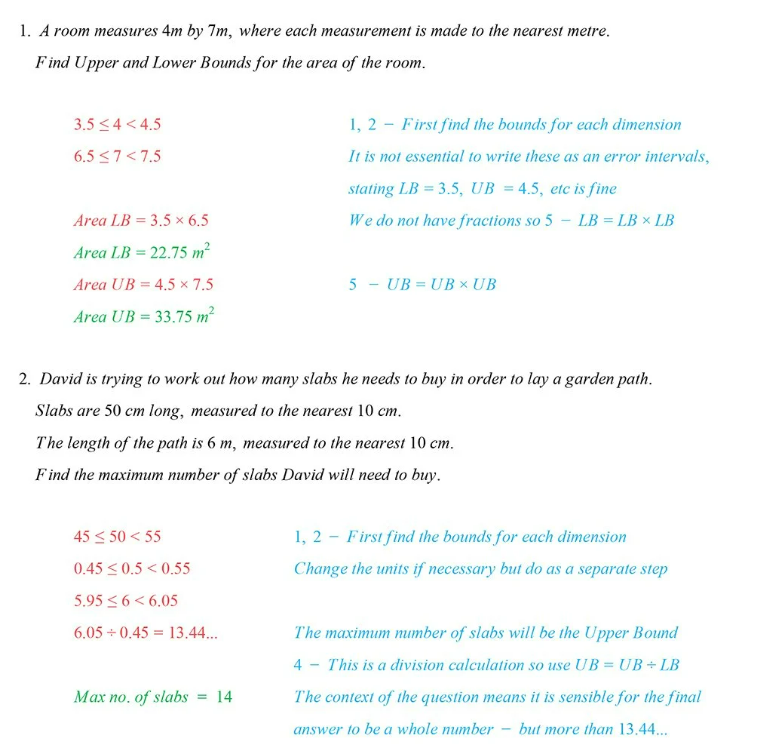# Edexcel IGCSE Maths复习笔记 1.12.2 Calculations using Bounds

Edexcel IGCSE Maths复习笔记 1.12.2 Calculations using Bounds

#### What are bounds?

• Bounds are the smallest – the Lower Bound (LB) – and largest – the Upper Bound (UB) – numbers that a rounded number can lie between

#### How do we find bounds?

• The basic rule is “Half Up, Half Down”
• More formally:
• UPPER BOUND – add on half the degree of accuracy
• LOWER BOUND – take off half the degree of accuracy
• ERROR INTERVAL: LB ≤ x < UB

#### Calculations using bounds

• Find bounds before calculating and then:
• For FRACTIONS/DIVISION:UB = UB ÷ LB and

LB = LB ÷ UB

• Otherwise:

UB = UB × UB etc.

Worked Example